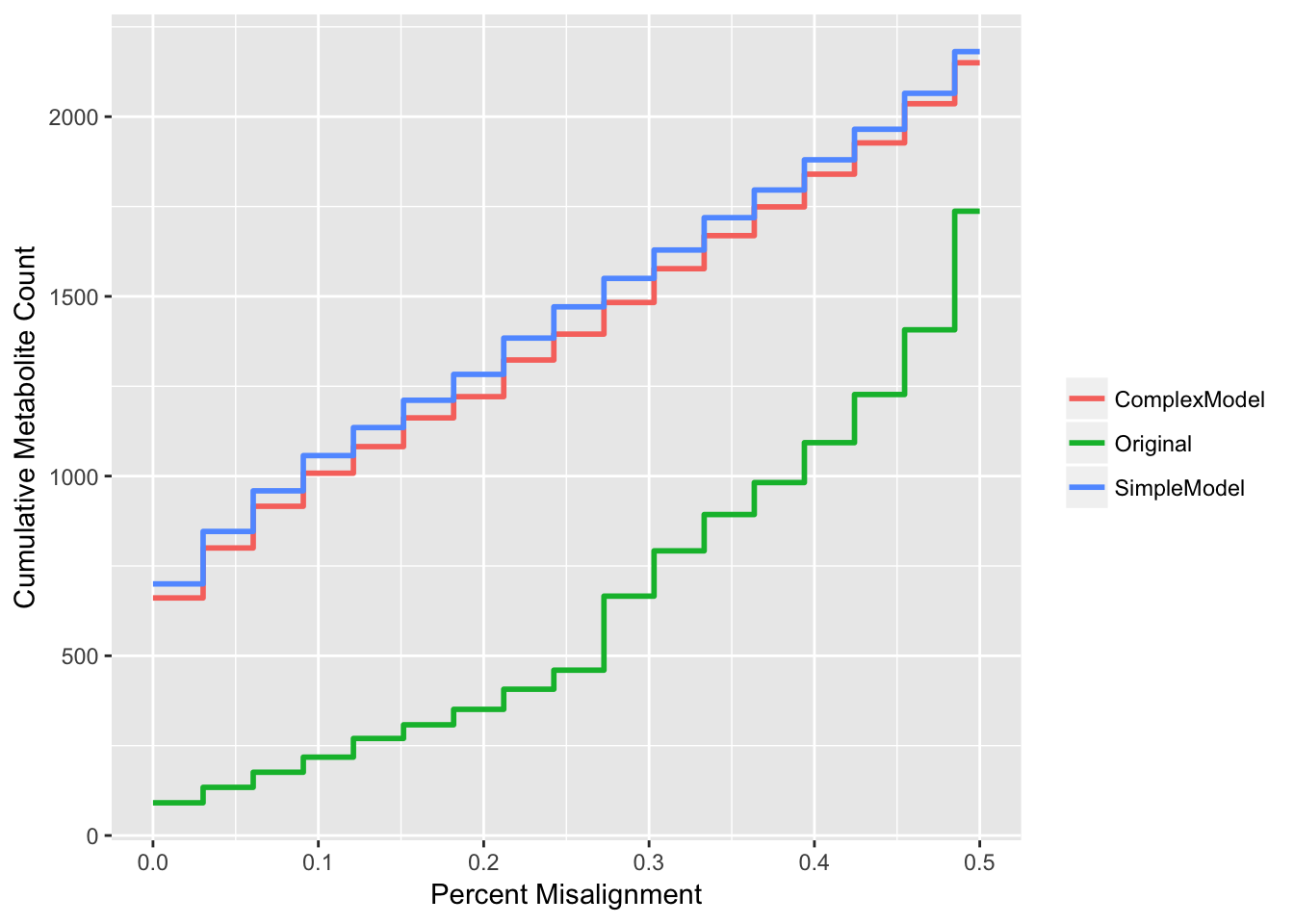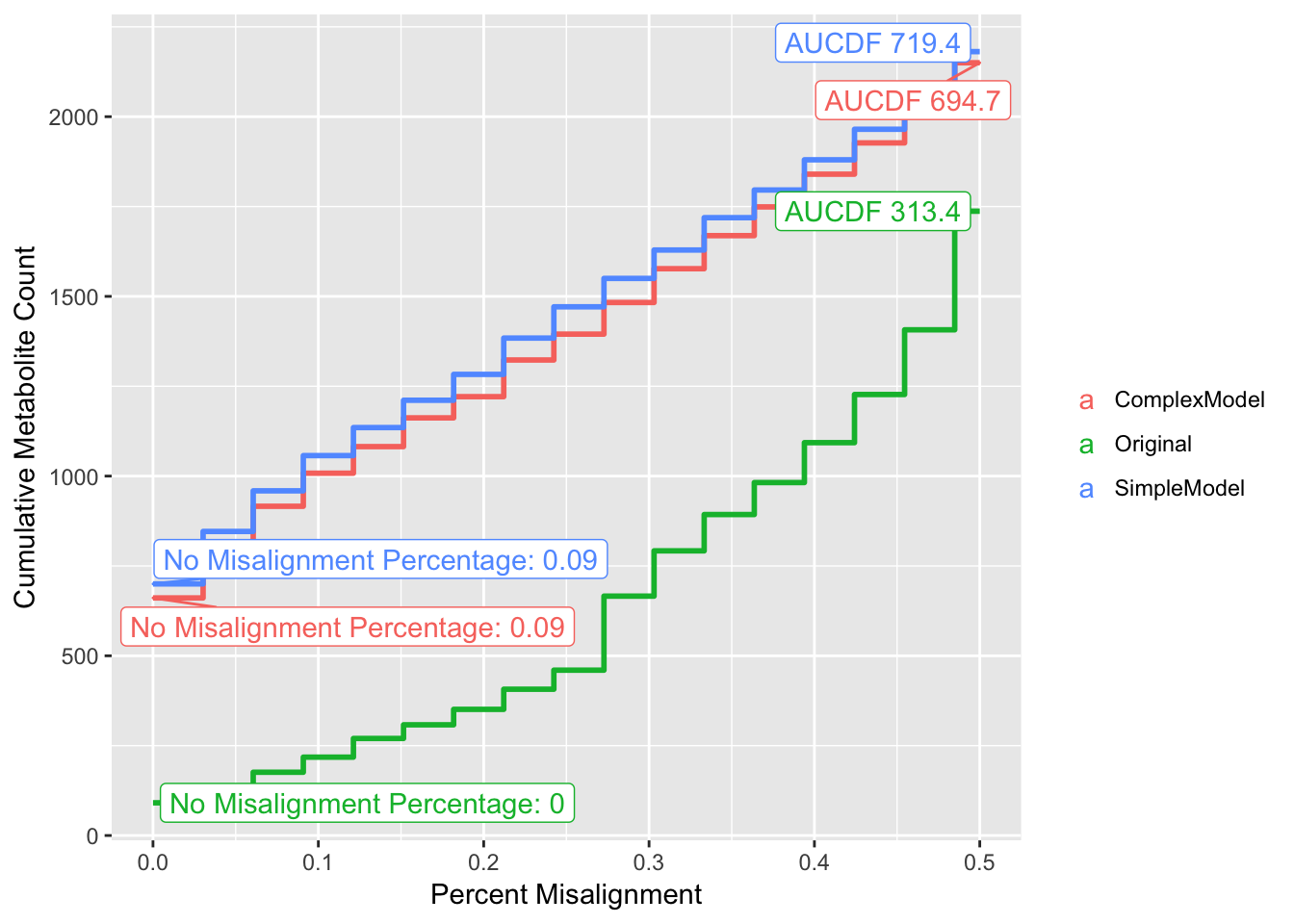citation: TBA

library(magrittr)
library(purrr)
library(dplyr)
library(ggplot2)
library(tidyr)

After applying our correction, we needed an approach to assess performance relative to baseline and other corrective models. There are many metrics of improvement that we could have considered. The number of perfectly aligned metabolites, the number of metabolites under some threshold of missingness across the sample, how well a certain cohort of metabolites improve, etc. In the end, we selected an approach that would take many of these metrics into consideration simultaneously.

We create some sample data here. Note that these data generated are highly representative of real data, in that they provide an accurate example of the structure of our data.

makeDset <- function() {
plate <-
1:10

well <-
1:10

metaboliteID <-
runif(10, 100, 900)

while (length(unique(metaboliteID)) != length(metaboliteID)) {
metaboliteID <-
runif(10, 100, 900)
}

dat <-
expand.grid(plate, well, metaboliteID) %>%
setNames(c('plate', 'well', 'metaboliteID'))

dat %<>%
mutate(plateWellID = paste0(plate, '_', well),
value = runif(n(), 1000, 1000000)) %>%
tbl_df()

return(dat)
}

d1 <- makeDset() %>%
mutate(method = 'Uncorrected')

d2 <- makeDset() %>%
mutate(method = 'Corrected')

dat <-
bind_rows(d1, d2)
dat
## # A tibble: 2,000 × 6
##    plate  well metaboliteID plateWellID    value      method
##    <int> <int>        <dbl>       <chr>    <dbl>       <chr>
## 1      1     1     166.7866         1_1 720824.4 Uncorrected
## 2      2     1     166.7866         2_1 464988.6 Uncorrected
## 3      3     1     166.7866         3_1 315725.1 Uncorrected
## 4      4     1     166.7866         4_1 306781.1 Uncorrected
## 5      5     1     166.7866         5_1 948846.8 Uncorrected
## 6      6     1     166.7866         6_1 527770.4 Uncorrected
## 7      7     1     166.7866         7_1 846891.3 Uncorrected
## 8      8     1     166.7866         8_1 849266.9 Uncorrected
## 9      9     1     166.7866         9_1 707627.8 Uncorrected
## 10    10     1     166.7866        10_1 310513.6 Uncorrected
## # ... with 1,990 more rows

For our sample data, the columns are:

• metaboliteID
• Numeric ID representing a unique measured metabolite.
• plateWellID
• Numeric ID representing a sample in which metabolites were measured.
• plate, well
• The plate and well that a sample was measured in.
• value
• The peak abundance measured in a sample.
• method
• We generated a dataset with the above columns for the uncorrected data and data after applying a correction algorithm. Those datasets were stacked togehter, with this column identifying the dataset each row belongs to.

Once again, this is data generated only to give an idea of what our data looks like. It is not used beyond this point.

Our samples were measured in plates of 96 wells. We said that a metabolite was misaligned for a plate if it is missing for all wells in that plate. For each metabolite, we calculated the percentage of plates that it was missing in, and then counted the number of metabolites at each value of % misalignment. We can then calculate a cumulative sum to get the number of metabolites that are X% misaligned or better. We also chose to only perform this calculation for up to 50% missingness, as using metabolites that were majority missing to evaluate performance of an alignment algorithm is impractical. After calculating these values, we have a CDF and we can calculate the area under the curve. The AUC was our most important metric in evaluating model performance.

# Perc Misaligned cdf -------------------------------------------------
maxPerc <- 0.5

misalign <-
dat %>%
# label any plates where a metaboliteID is totally missing
group_by(method, metaboliteID, plate) %>%
summarise(misaligned = all(value == 0)) %>%
ungroup() %>%
# Calculate % of plates totally missing
group_by(method, metaboliteID) %>%
summarise(misaligned = mean(misaligned)) %>%
ungroup() %>%
# Count the number of metaboliteIDs that are at any given misalignment %
group_by(method, misaligned) %>%
summarise(n = n()) %>%
ungroup() %>%
# Only include metabolites that are 50% missing or less
filter(misaligned <= maxPerc) %>%
# Calculate the cumulative count of metabolites that are 5% misaligned or better.
group_by(method) %>%
mutate(cumMisaligned = cumsum(n)) %>%
ungroup()

misalign
## # A tibble: 51 × 4
##          method misaligned     n cumMisaligned
##           <chr>      <dbl> <int>         <int>
## 1  ComplexModel 0.00000000   661           661
## 2  ComplexModel 0.03030303   139           800
## 3  ComplexModel 0.06060606   116           916
## 4  ComplexModel 0.09090909    92          1008
## 5  ComplexModel 0.12121212    74          1082
## 6  ComplexModel 0.15151515    80          1162
## 7  ComplexModel 0.18181818    59          1221
## 8  ComplexModel 0.21212121   102          1323
## 9  ComplexModel 0.24242424    72          1395
## 10 ComplexModel 0.27272727    88          1483
## # ... with 41 more rows

Here we calculate the area under our CDF by multiplying the width and height of each ‘step’ in the CDF and summing them together. We format it with x and y values so it can be added as a label to our plot later.

auc <-
misalign %>%
group_by(method) %>%
mutate(width = c(diff(misaligned), maxPerc - max(misaligned))) %>%
mutate(auc = width * cumMisaligned) %>%
summarise(auc = sum(auc),
y = max(cumMisaligned)) %>%
mutate(label = paste('AUCDF', round(auc, 1)),
x = maxPerc) %>%
select(method, label, x, y)

auc
## # A tibble: 3 × 4
##         method       label     x     y
##          <chr>       <chr> <dbl> <int>
## 1 ComplexModel AUCDF 694.7   0.5  2150
## 2     Original AUCDF 313.4   0.5  1737
## 3  SimpleModel AUCDF 719.4   0.5  2181

Though AUC is our most important metric, we also consider the number of metabolites that have no missing plates. We calculate and format for labeling plots later here.

nMetabolites <-
dat %>%
group_by(method) %>%
summarise(nMetabolite = length(unique(metaboliteID)))

perfaligned <-
misalign %>%
left_join(nMetabolites, by = 'method') %>%
filter(misaligned == 0) %>%
group_by(method) %>%
summarise(label = cumMisaligned / nMetabolite,
naligned = label * nMetabolite,
x = -0.00,
y = naligned) %>%
mutate() %>%
mutate(label = paste('No Misalignment Percentage:', round(label, 2))) %>%
ungroup() %>%
select(method, label, x, y)

perfaligned
## # A tibble: 3 × 4
##         method                            label     x     y
##          <chr>                            <chr> <dbl> <dbl>
## 1 ComplexModel No Misalignment Percentage: 0.09     0   661
## 2     Original    No Misalignment Percentage: 0     0    91
## 3  SimpleModel No Misalignment Percentage: 0.09     0   700

The CDF step function needs an additional value at the end so that it ends on a horizontal segment, not a vertical one. That is that the ‘tail’ is for.

cdftails <-
misalign %>%
group_by(method) %>%
summarise(cumMisaligned = max(cumMisaligned)) %>%
mutate(misaligned = maxPerc)

misalign %<>% full_join(cdftails, by = c("method", "misaligned", "cumMisaligned"))

Now we plot, one with labels, and one without to make it easier to see the graph.

plotText <-
rbind(perfaligned, auc)

p <-
misalign %>%
ggplot(aes(colour = method)) +
geom_step(aes(x = misaligned, y = cumMisaligned), direction = 'hv', size = 1) +
ylab('Cumulative Metabolite Count') +
xlab('Percent Misalignment') +
scale_colour_discrete('')

pp +
ggrepel::geom_label_repel(data = plotText, aes(label = label, x = x, y = y))We see from our graphs that the data corrected with the simpler model, and with the more complex model, both outperform the uncorrected data. There are many more metabolites that are not missing across plates, and many more metabolites that are 50% misaligned or less. The simpler and more complex models seem to have very similar performance overall, with the simpler model having slightly greater AUC. In this case, we would elect to use the more parsimonious model going forward.